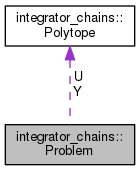fmrbenchmark  0.0.4
integrator_chains::Problem Class Reference

Representation of problem instances. More...

`#include <problem.hpp>`

Collaboration diagram for integrator_chains::Problem:[legend]

## Public Member Functions

bool change_order (int new_numdim_output, int new_highest_order_deriv)

std::string dumpJSON () const
Get description of problem in JSON. More...

int get_highest_order_deriv () const

int get_numdim_output () const

bool is_consistent () const

void to_formula (std::ostream &out) const
Create formula using SPIN LTL syntax http://spinroot.com/spin/Man/ltl.html. More...

## Static Public Member Functions

static Problemrandom (const Eigen::Vector2i &numdim_output_bounds, const Eigen::Vector2i &highest_order_deriv_bounds, const Eigen::VectorXd &Y_box, const Eigen::VectorXd &U_box, const Eigen::Vector2i &number_goals_bounds, const Eigen::Vector2i &number_obstacles_bounds, const Eigen::Vector2d &period_bounds, const Eigen::VectorXd &Xinit_bounds=Eigen::VectorXd())
Generate a random problem instance. More...

## Public Attributes

std::vector< LabeledPolytope * > goals

std::vector< LabeledPolytope * > obstacles

double period

PolytopeU

Eigen::VectorXd Xinit

PolytopeY

## Friends

std::ostream & operator<< (std::ostream &out, const Problem &prob)
Output description of problem in JSON to a stream.

## Detailed Description

Representation of problem instances.

## Member Function Documentation

 std::string integrator_chains::Problem::dumpJSON ( ) const

Get description of problem in JSON.

 Problem * integrator_chains::Problem::random ( const Eigen::Vector2i & numdim_output_bounds, const Eigen::Vector2i & highest_order_deriv_bounds, const Eigen::VectorXd & Y_box, const Eigen::VectorXd & U_box, const Eigen::Vector2i & number_goals_bounds, const Eigen::Vector2i & number_obstacles_bounds, const Eigen::Vector2d & period_bounds, const Eigen::VectorXd & Xinit_bounds = `Eigen::VectorXd()` )
static

Generate a random problem instance.

Parameters
 numdim_output_bounds range of integers from which the dimension of the output space will be chosen. highest_order_deriv_bounds range of integers from which the order of derivation (i.e., the number of integrators in the ODE defining the system) will be chosen. Y_box the compact subset of the output space in which the trajectory must remain, defined using the same format as for Polytope::box(). Unlike most other parameters of this method, `Y_box` is not a definition of the support of a probability density function. However, the number of elements in it that are actually used depends on the randomly chosen number of dimensions of the output space. U_box analogous to `Y_box` but for the input space. number_goals_bounds range of integers from which the number of goal polytopes in the output space will be chosen. number_obstacles_bounds analogous to `number_goals_bounds` but for obstacles. period_bounds range from which is uniformly randomly chosen the constant period by which the original system is discretized. Xinit_bounds (optional) the box from which the initial state will be chosen. It is assumed to be consistent with `Y_box`. Bounds for missing dimensions are assumed to be [0,0], i.e., Xinit is assigned 0.0 at the corresponding indices. E.g., if Xinit_bounds has size 0 or is not given, then Xinit is the origin of the state space.

N.B., several parameters depend on each other in terms of consistency. E.g., if `numdim_output_bounds` = [2, 3], then Y_box must have length of at least 6 (cf. documentation for Polytope::box()).

This method relies on rand() for pseudo-randomness and assumes that something else has seeded the RNG.

 void integrator_chains::Problem::to_formula ( std::ostream & out ) const

Create formula using SPIN LTL syntax http://spinroot.com/spin/Man/ltl.html.

Support for other syntax is coming soon.

The documentation for this class was generated from the following file:
• integrator_chains/dynamaestro/include/problem.hpp